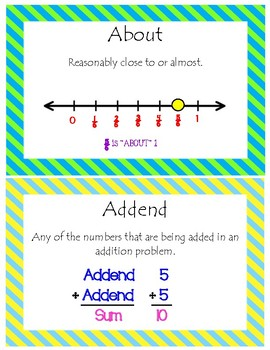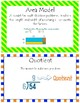# 82 3rd Grade Word Wall VocabularySubject
Resource Type
File Type

PDF

(10 MB|41 pages)
Product Description
Word Wall Cards with term, definition and visual.

Words for entire school year based on Engage NY curriculum.

Each border is different.

Words Included Are:
Vocabulary Included:

analog clock
area
area model
array
attribute
bar graph
capacity
centimeter
commutative property
compose
continuous
data
denominator
diagonal -
difference digital clock
distributive property
divide/division
endpoint
equal groups
equation
equivalent fractions
estimate
expression
factor
fractional unit
gram
halfway
horizontal
interval
key
kilogram
line plot
liquid volume
liter
meter
milliliter
multiplication/multiply
non-unit fraction
number bond number
number sentence
numerator
parallel
parallelogram
parentheses
perimeter
picture graph
place value chart
plot
point
polygon
product
quotient
reasonable
rectangle
regular polygon
rhombus
right angle
rotate
round
row/column
scale
seconds
square
square unit
survey
tape diagram
tick marks
trapezoid
unit
unit fraction
unit square
unknown
vertical
whole number
Total Pages
41 pages
Does not apply
Teaching Duration
N/A
Report this Resource to TpT
Reported resources will be reviewed by our team. Report this resource to let us know if this resource violates TpT’s content guidelines.